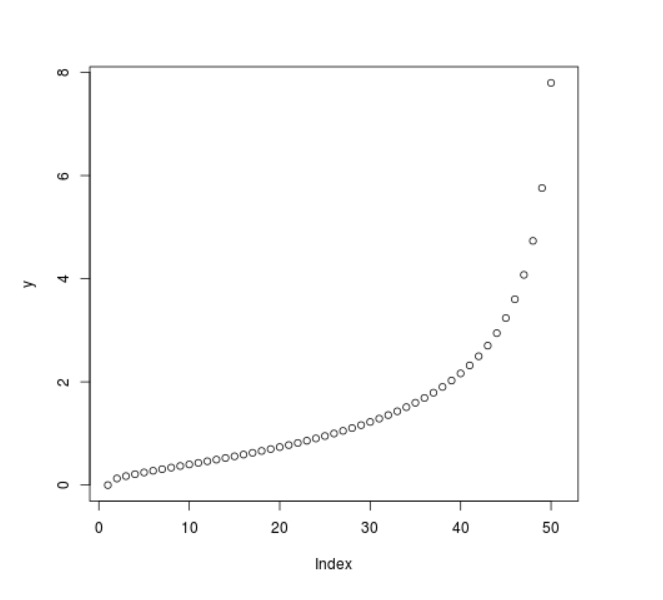Related Articles
Compute value of Log Normal Quantile Function in R Programming – qlnorm() Function
• Last Updated : 25 Jun, 2020

`qlnorm()` function in R Language is used to compute the value of log normal quantile function. It also creates a plot of the quantile function of log normal distribution.

Syntax: qlnorm(vec)

Parameters:
vec: x-values for normal density

Example 1:

 `# R program to compute value of ` `# log normal quantile function ` ` `  `# Creating x-values for density ` `x <``-` `seq(``0``, ``1``, by ``=` `0.2``) ` ` `  `# Calling qlnorm() function ` `y <``-` `qlnorm(x) ` `y `

Output:

``` 0.0000000 0.4310112 0.7761984 1.2883304 2.3201254       Inf
```

Example 2:

 `# R program to compute value of ` `# log normal quantile function ` ` `  `# Creating x-values for density ` `x <``-` `seq(``0``, ``1``, by ``=` `0.02``) ` ` `  `# Calling qlnorm() function ` `y <``-` `qlnorm(x) ` ` `  `# Plot a graph ` `plot(y) `

Output:My Personal Notes arrow_drop_up
Recommended Articles
Page :